## 4.5 Relative Motion in One and Two Dimensions

### Learning Objectives

By the end of this section, you will be able to:

• Explain the concept of reference frames.
• Write the position and velocity vector equations for relative motion.
• Draw the position and velocity vectors for relative motion.
• Analyze one-dimensional and two-dimensional relative motion problems using the position and velocity vector equations.

Motion does not happen in isolation. If you’re riding in a train moving at 10 m/s east, this velocity is measured relative to the ground on which you’re traveling. However, if another train passes you at 15 m/s east, your velocity relative to this other train is different from your velocity relative to the ground. Your velocity relative to the other train is 5 m/s west. To explore this idea further, we first need to establish some terminology.

### Reference Frames

To discuss relative motion in one or more dimensions, we first introduce the concept of reference frames. When we say an object has a certain velocity, we must state it has a velocity with respect to a given reference frame. In most examples we have examined so far, this reference frame has been Earth. If you say a person is sitting in a train moving at 10 m/s east, then you imply the person on the train is moving relative to the surface of Earth at this velocity, and Earth is the reference frame. We can expand our view of the motion of the person on the train and say Earth is spinning in its orbit around the Sun, in which case the motion becomes more complicated. In this case, the solar system is the reference frame. In summary, all discussion of relative motion must define the reference frames involved. We now develop a method to refer to reference frames in relative motion.

### Relative Motion in One Dimension

We introduce relative motion in one dimension first, because the velocity vectors simplify to having only two possible directions. Take the example of the person sitting in a train moving east. If we choose east as the positive direction and Earth as the reference frame, then we can write the velocity of the train with respect to the Earth as ${\overset{\to }{v}}_{\text{TE}}=10\,\text{m}\text{/}\text{s}\,\hat{i}$ east, where the subscripts TE refer to train and Earth. Let’s now say the person gets up out of /her seat and walks toward the back of the train at 2 m/s. This tells us she has a velocity relative to the reference frame of the train. Since the person is walking west, in the negative direction, we write her velocity with respect to the train as ${\overset{\to }{v}}_{\text{PT}}=-2\,\text{m}\text{/}\text{s}\,\hat{i}\text{.}$ We can add the two velocity vectors to find the velocity of the person with respect to Earth. This relative velocity is written as

${\overset{\to }{v}}_{\text{PE}}={\overset{\to }{v}}_{\text{PT}}+{\overset{\to }{v}}_{\text{TE}}.$

Note the ordering of the subscripts for the various reference frames in (Figure). The subscripts for the coupling reference frame, which is the train, appear consecutively in the right-hand side of the equation. (Figure) shows the correct order of subscripts when forming the vector equation.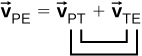Figure 4.24 When constructing the vector equation, the subscripts for the coupling reference frame appear consecutively on the inside. The subscripts on the left-hand side of the equation are the same as the two outside subscripts on the right-hand side of the equation.

Adding the vectors, we find ${\overset{\to }{v}}_{\text{PE}}=8\,\text{m}\text{/}\text{s}\,\hat{i}\text{,}$ so the person is moving 8 m/s east with respect to Earth. Graphically, this is shown in (Figure).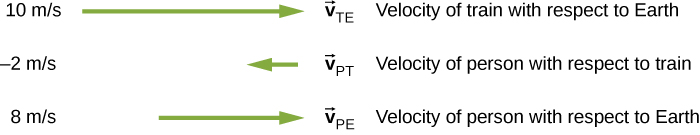Figure 4.25 Velocity vectors of the train with respect to Earth, person with respect to the train, and person with respect to Earth.

### Relative Velocity in Two Dimensions

We can now apply these concepts to describing motion in two dimensions. Consider a particle P and reference frames S and ${S}^{\prime },$ as shown in (Figure). The position of the origin of ${S}^{\prime }$ as measured in S is ${\overset{\to }{r}}_{{S}^{\prime }S},$ the position of P as measured in ${S}^{\prime }$ is ${\overset{\to }{r}}_{P{S}^{\prime }},$ and the position of P as measured in S is ${\overset{\to }{r}}_{PS}.$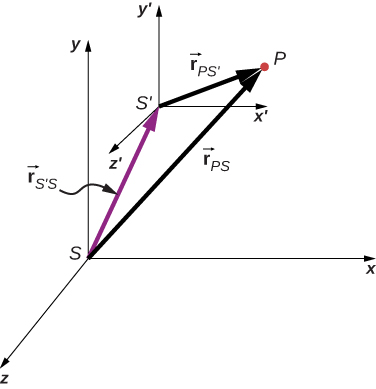Figure 4.26 The positions of particle P relative to frames S and ${S}^{\prime }$ are ${\overset{\to }{r}}_{PS}$ and ${\overset{\to }{r}}_{P{S}^{\prime }},$ respectively.

From (Figure) we see that

${\overset{\to }{r}}_{PS}={\overset{\to }{r}}_{P{S}^{\prime }}+{\overset{\to }{r}}_{{S}^{\prime }S}.$

The relative velocities are the time derivatives of the position vectors. Therefore,

${\overset{\to }{v}}_{PS}={\overset{\to }{v}}_{P{S}^{\prime }}+{\overset{\to }{v}}_{{S}^{\prime }S}.$

The velocity of a particle relative to S is equal to its velocity relative to${S}^{\prime }$plus the velocity of${S}^{\prime }$relative to S.

We can extend (Figure) to any number of reference frames. For particle P with velocities ${\overset{\to }{v}}_{PA},{\overset{\to }{v}}_{PB}\text{,}\,\text{and}\,{\overset{\to }{v}}_{PC}$ in frames A, B, and C,

${\overset{\to }{v}}_{PC}={\overset{\to }{v}}_{PA}+{\overset{\to }{v}}_{AB}+{\overset{\to }{v}}_{BC}.$

We can also see how the accelerations are related as observed in two reference frames by differentiating (Figure):

${\overset{\to }{a}}_{PS}={\overset{\to }{a}}_{P{S}^{\prime }}+{\overset{\to }{a}}_{{S}^{\prime }S}.$

We see that if the velocity of ${S}^{\prime }$ relative to S is a constant, then ${\overset{\to }{a}}_{{S}^{\prime }S}=0$ and

${\overset{\to }{a}}_{PS}={\overset{\to }{a}}_{P{S}^{\prime }}.$

This says the acceleration of a particle is the same as measured by two observers moving at a constant velocity relative to each other.

### Example

#### Motion of a Car Relative to a Truck

A truck is traveling south at a speed of 70 km/h toward an intersection. A car is traveling east toward the intersection at a speed of 80 km/h ((Figure)). What is the velocity of the car relative to the truck?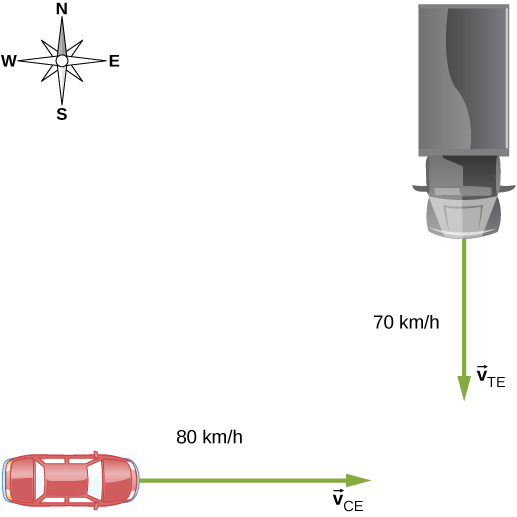Figure 4.27 A car travels east toward an intersection while a truck travels south toward the same intersection.

#### Strategy

First, we must establish the reference frame common to both vehicles, which is Earth. Then, we write the velocities of each with respect to the reference frame of Earth, which enables us to form a vector equation that links the car, the truck, and Earth to solve for the velocity of the car with respect to the truck.

#### Solution

The velocity of the car with respect to Earth is ${\overset{\to }{v}}_{\text{CE}}=80\,\text{km}\text{/}\text{h}\,\hat{i}.$ The velocity of the truck with respect to Earth is ${\overset{\to }{v}}_{\text{TE}}=-70\,\text{km}\text{/}\text{h}\,\hat{j}\text{.}$ Using the velocity addition rule, the relative motion equation we are seeking is

${\overset{\to }{v}}_{\text{CT}}={\overset{\to }{v}}_{\text{CE}}+{\overset{\to }{v}}_{\text{ET}}.$

Here, ${\overset{\to }{v}}_{\text{CT}}$ is the velocity of the car with respect to the truck, and Earth is the connecting reference frame. Since we have the velocity of the truck with respect to Earth, the negative of this vector is the velocity of Earth with respect to the truck: ${\overset{\to }{v}}_{\text{ET}}=\text{−}{\overset{\to }{v}}_{\text{TE}}.$ The vector diagram of this equation is shown in (Figure).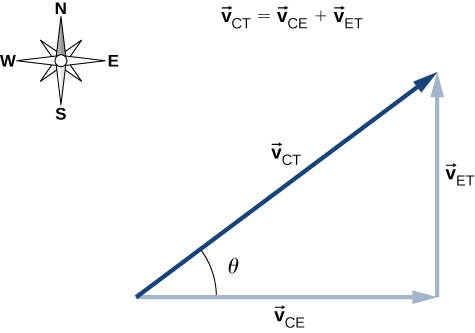Figure 4.28 Vector diagram of the vector equation ${\overset{\to }{v}}_{\text{CT}}={\overset{\to }{v}}_{\text{CE}}+{\overset{\to }{v}}_{\text{ET}}$.

We can now solve for the velocity of the car with respect to the truck:

$|{\overset{\to }{v}}_{\text{CT}}|=\sqrt{(80.0\,\text{km}\text{/}{\text{h})}^{2}+(70.0\,\text{km}\text{/}{\text{h})}^{2}}=106.\,\text{km}\text{/}\text{h}$

and

$\theta ={\text{tan}}^{-1}(\frac{70.0}{80.0})=41.2\text{°}\,\text{north of east.}$

#### Significance

Drawing a vector diagram showing the velocity vectors can help in understanding the relative velocity of the two objects.

A boat heads north in still water at 4.5 m/s directly across a river that is running east at 3.0 m/s. What is the velocity of the boat with respect to Earth?

### Example

#### Flying a Plane in a Wind

A pilot must fly his plane due north to reach his destination. The plane can fly at 300 km/h in still air. A wind is blowing out of the northeast at 90 km/h. (a) What is the speed of the plane relative to the ground? (b) In what direction must the pilot head her plane to fly due north?

#### Strategy

The pilot must point her plane somewhat east of north to compensate for the wind velocity. We need to construct a vector equation that contains the velocity of the plane with respect to the ground, the velocity of the plane with respect to the air, and the velocity of the air with respect to the ground. Since these last two quantities are known, we can solve for the velocity of the plane with respect to the ground. We can graph the vectors and use this diagram to evaluate the magnitude of the plane’s velocity with respect to the ground. The diagram will also tell us the angle the plane’s velocity makes with north with respect to the air, which is the direction the pilot must head her plane.

#### Solution

The vector equation is ${\overset{\to }{v}}_{\text{PG}}={\overset{\to }{v}}_{\text{PA}}+{\overset{\to }{v}}_{\text{AG}},$ where P = plane, A = air, and G = ground. From the geometry in (Figure), we can solve easily for the magnitude of the velocity of the plane with respect to the ground and the angle of the plane’s heading, $\theta .$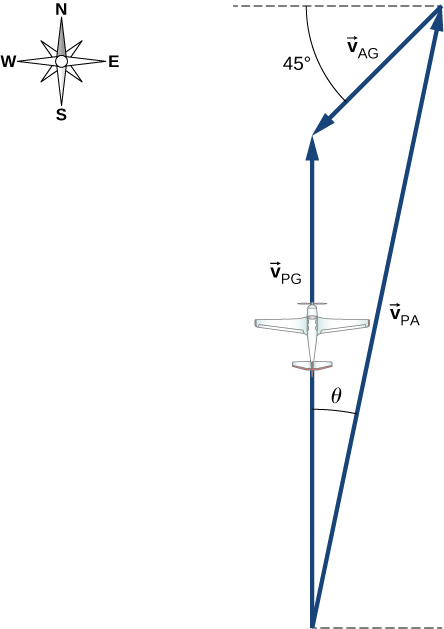Figure 4.29 Vector diagram for (Figure) showing the vectors ${\overset{\to }{v}}_{\text{PA}}\text{,}\,{\overset{\to }{v}}_{\text{AG}},\,\text{and}\,\,{\overset{\to }{v}}_{\text{PG}}.$

(a) Known quantities:

$|{\overset{\to }{v}}_{\text{PA}}|=300\,\text{km}\text{/}\text{h}$
$|{\overset{\to }{v}}_{\text{AG}}|=90\,\text{km}\text{/}\text{h}$

Substituting into the equation of motion, we obtain $|{\overset{\to }{v}}_{\text{PG}}|=230\,\text{km}\text{/}\text{h}\text{.}$

(b) The angle $\theta ={\text{tan}}^{-1}\frac{63.64}{300}=12\text{°}$ east of north.

### Summary

• When analyzing motion of an object, the reference frame in terms of position, velocity, and acceleration needs to be specified.
• Relative velocity is the velocity of an object as observed from a particular reference frame, and it varies with the choice of reference frame.
• If S and ${S}^{\prime }$ are two reference frames moving relative to each other at a constant velocity, then the velocity of an object relative to S is equal to its velocity relative to ${S}^{\prime }$ plus the velocity of ${S}^{\prime }$ relative to S.
• If two reference frames are moving relative to each other at a constant velocity, then the accelerations of an object as observed in both reference frames are equal.

### Key Equations

 Position vector $\overset{\to }{r}(t)=x(t)\hat{i}+y(t)\hat{j}+z(t)\hat{k}$ Displacement vector $\text{Δ}\overset{\to }{r}=\overset{\to }{r}({t}_{2})-\overset{\to }{r}({t}_{1})$ Velocity vector $\overset{\to }{v}(t)=\underset{\text{Δ}t\to 0}{\text{lim}}\frac{\overset{\to }{r}(t+\text{Δ}t)-\overset{\to }{r}(t)}{\text{Δ}t}=\frac{d\overset{\to }{r}}{dt}$ Velocity in terms of components $\overset{\to }{v}(t)={v}_{x}(t)\hat{i}+{v}_{y}(t)\hat{j}+{v}_{z}(t)\hat{k}$ Velocity components ${v}_{x}(t)=\frac{dx(t)}{dt}\enspace{v}_{y}(t)=\frac{dy(t)}{dt}\enspace{v}_{z}(t)=\frac{dz(t)}{dt}$ Average velocity ${\overset{\to }{v}}_{\text{avg}}=\frac{\overset{\to }{r}({t}_{2})-\overset{\to }{r}({t}_{1})}{{t}_{2}-{t}_{1}}$ Instantaneous acceleration $\overset{\to }{a}(t)=\underset{t\to 0}{\text{lim}}\frac{\overset{\to }{v}(t+\text{Δ}t)-\overset{\to }{v}(t)}{\text{Δ}t}=\frac{d\overset{\to }{v}(t)}{dt}$ Instantaneous acceleration, component form $\overset{\to }{a}(t)=\frac{d{v}_{x}(t)}{dt}\hat{i}+\frac{d{v}_{y}(t)}{dt}\hat{j}+\frac{d{v}_{z}(t)}{dt}\hat{k}$ Instantaneous acceleration as second derivatives of position $\overset{\to }{a}(t)=\frac{{d}^{2}x(t)}{d{t}^{2}}\hat{i}+\frac{{d}^{2}y(t)}{d{t}^{2}}\hat{j}+\frac{{d}^{2}z(t)}{d{t}^{2}}\hat{k}$ Time of flight ${T}_{\text{tof}}=\frac{2({v}_{0}\text{sin}\theta )}{g}$ Trajectory $y=(\text{tan}{\theta }_{0})x-[\frac{g}{2{({v}_{0}\text{cos}{\theta }_{0})}^{2}}]{x}^{2}$ Range $R=\frac{{v}_{0}^{2}\text{sin}\,2{\theta }_{0}}{g}$ Centripetal acceleration ${a}_{\text{C}}=\frac{{v}^{2}}{r}$ Position vector, uniform circular motion $\overset{\to }{r}(t)=A\text{cos}\,\omega t\,\hat{i}+A\,\text{sin}\,\omega t\hat{j}$ Velocity vector, uniform circular motion $\overset{\to }{v}(t)=\frac{d\overset{\to }{r}(t)}{dt}=\text{−}A\omega \,\text{sin}\,\omega t\hat{i}+A\omega \,\text{cos}\,\omega t\hat{j}$ Acceleration vector, uniform circular motion $\overset{\to }{a}(t)=\frac{d\overset{\to }{v}(t)}{dt}=\text{−}A{\omega }^{2}\,\text{cos}\,\omega t\hat{i}-A{\omega }^{2}\,\text{sin}\,\omega t\hat{j}$ Tangential acceleration ${a}_{\text{T}}=\frac{d|\overset{\to }{v}|}{dt}$ Total acceleration $\overset{\to }{a}={\overset{\to }{a}}_{\text{C}}+{\overset{\to }{a}}_{\text{T}}$ Position vector in frame S is the position vector in frame ${S}^{\prime }$ plus the vector from the origin of S to the origin of ${S}^{\prime }$ ${\overset{\to }{r}}_{PS}={\overset{\to }{r}}_{P{S}^{\prime }}+{\overset{\to }{r}}_{{S}^{\prime }S}$ Relative velocity equation connecting two reference frames ${\overset{\to }{v}}_{PS}={\overset{\to }{v}}_{P{S}^{\prime }}+{\overset{\to }{v}}_{{S}^{\prime }S}$ Relative velocity equation connecting more than two reference frames ${\overset{\to }{v}}_{PC}={\overset{\to }{v}}_{PA}+{\overset{\to }{v}}_{AB}+{\overset{\to }{v}}_{BC}$ Relative acceleration equation ${\overset{\to }{a}}_{PS}={\overset{\to }{a}}_{P{S}^{\prime }}+{\overset{\to }{a}}_{{S}^{\prime }S}$

### Conceptual Questions

What frame or frames of reference do you use instinctively when driving a car? When flying in a commercial jet?

A basketball player dribbling down the court usually keeps his eyes fixed on the players around him. He is moving fast. Why doesn’t he need to keep his eyes on the ball?

If someone is riding in the back of a pickup truck and throws a softball straight backward, is it possible for the ball to fall straight down as viewed by a person standing at the side of the road? Under what condition would this occur? How would the motion of the ball appear to the person who threw it?

The hat of a jogger running at constant velocity falls off the back of his head. Draw a sketch showing the path of the hat in the jogger’s frame of reference. Draw its path as viewed by a stationary observer. Neglect air resistance.

A clod of dirt falls from the bed of a moving truck. It strikes the ground directly below the end of the truck. (a) What is the direction of its velocity relative to the truck just before it hits? (b) Is this the same as the direction of its velocity relative to ground just before it hits? Explain your answers.

### Problems

The coordinate axes of the reference frame ${S}^{\prime }$ remain parallel to those of S, as ${S}^{\prime }$ moves away from S at a constant velocity ${\overset{\to }{v}}_{{\text{S}}^{\prime }}=(4.0\hat{i}+3.0\hat{j}+5.0\hat{k})\,\text{m}\text{/}\text{s}.$ (a) If at time t = 0 the origins coincide, what is the position of the origin ${O}^{\prime }$ in the S frame as a function of time? (b) How is particle position for $\overset{\to }{r}(t)$ and ${\overset{\to }{r}}^{\prime }(t),$ as measured in S and ${S}^{\prime },$ respectively, related? (c) What is the relationship between particle velocities $\overset{\to }{v}(t)\,\text{and}\,{\overset{\to }{v}}^{\prime }(t)?$ (d) How are accelerations $\overset{\to }{a}\text{(t)}\,\text{and}\,{\overset{\to }{a}}^{\prime }\text{(t)}$ related?

The coordinate axes of the reference frame ${S}^{\prime }$ remain parallel to those of S, as ${S}^{\prime }$ moves away from S at a constant velocity ${\overset{\to }{v}}_{{S}^{\prime }S}=(1.0\hat{i}+2.0\hat{j}+3.0\hat{k})t\,\text{m}\text{/}\text{s}$. (a) If at time t = 0 the origins coincide, what is the position of origin ${O}^{\prime }$ in the S frame as a function of time? (b) How is particle position for $\overset{\to }{r}(t)$ and ${\overset{\to }{r}}^{\prime }(t)$, as measured in S and ${S}^{\prime },$ respectively, related? (c) What is the relationship between particle velocities $\overset{\to }{v}(t)\,\text{and}\,{\overset{\to }{v}}^{\prime }(t)?$ (d) How are accelerations $\overset{\to }{a}\text{(t)� and}\,{\overset{\to }{a}}^{\prime }\text{(t)}$ related?

The velocity of a particle in reference frame A is $(2.0\hat{i}+3.0\hat{j}\text{)}\,\text{m}\text{/}\text{s}.$ The velocity of reference frame A with respect to reference frame B is $4.0\hat{k}\text{m}\text{/}\text{s},$ and the velocity of reference frame B with respect to C is $2.0\hat{j}\text{m}\text{/}\text{s}.$ What is the velocity of the particle in reference frame C?

Raindrops fall vertically at 4.5 m/s relative to the earth. What does an observer in a car moving at 22.0 m/s in a straight line measure as the velocity of the raindrops?

A seagull can fly at a velocity of 9.00 m/s in still air. (a) If it takes the bird 20.0 min to travel 6.00 km straight into an oncoming wind, what is the velocity of the wind? (b) If the bird turns around and flies with the wind, how long will it take the bird to return 6.00 km?

A ship sets sail from Rotterdam, heading due north at 7.00 m/s relative to the water. The local ocean current is 1.50 m/s in a direction $40.0\text{°}$ north of east. What is the velocity of the ship relative to Earth?

A boat can be rowed at 8.0 km/h in still water. (a) How much time is required to row 1.5 km downstream in a river moving 3.0 km/h relative to the shore? (b) How much time is required for the return trip? (c) In what direction must the boat be aimed to row straight across the river? (d) Suppose the river is 0.8 km wide. What is the velocity of the boat with respect to Earth and how much time is required to get to the opposite shore? (e) Suppose, instead, the boat is aimed straight across the river. How much time is required to get across and how far downstream is the boat when it reaches the opposite shore?

A small plane flies at 200 km/h in still air. If the wind blows directly out of the west at 50 km/h, (a) in what direction must the pilot head her plane to move directly north across land and (b) how long does it take her to reach a point 300 km directly north of her starting point?

A cyclist traveling southeast along a road at 15 km/h feels a wind blowing from the southwest at 25 km/h. To a stationary observer, what are the speed and direction of the wind?

A river is moving east at 4 m/s. A boat starts from the dock heading $30\text{°}$ north of west at 7 m/s. If the river is 1800 m wide, (a) what is the velocity of the boat with respect to Earth and (b) how long does it take the boat to cross the river?

A Formula One race car is traveling at 89.0 m/s along a straight track enters a turn on the race track with radius of curvature of 200.0 m. What centripetal acceleration must the car have to stay on the track?

A particle travels in a circular orbit of radius 10 m. Its speed is changing at a rate of $15.0\,\text{m}\text{/}{\text{s}}^{2}$ at an instant when its speed is 40.0 m/s. What is the magnitude of the acceleration of the particle?

The driver of a car moving at 90.0 km/h presses down on the brake as the car enters a circular curve of radius 150.0 m. If the speed of the car is decreasing at a rate of 9.0 km/h each second, what is the magnitude of the acceleration of the car at the instant its speed is 60.0 km/h?

A race car entering the curved part of the track at the Daytona 500 drops its speed from 85.0 m/s to 80.0 m/s in 2.0 s. If the radius of the curved part of the track is 316.0 m, calculate the total acceleration of the race car at the beginning and ending of reduction of speed.

An elephant is located on Earth’s surface at a latitude $\lambda .$ Calculate the centripetal acceleration of the elephant resulting from the rotation of Earth around its polar axis. Express your answer in terms of $\lambda ,$ the radius ${R}_{E}$ of Earth, and time T for one rotation of Earth. Compare your answer with g for $\lambda =40\text{°}.$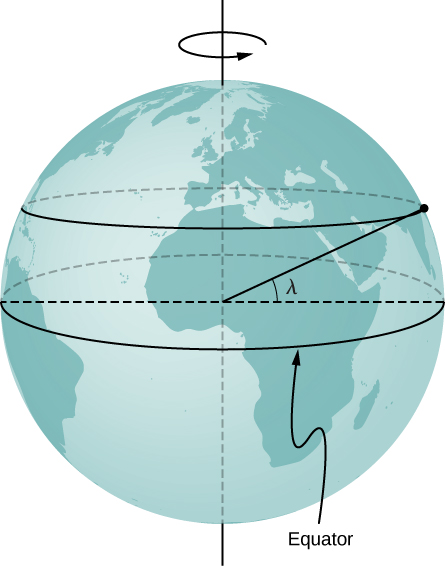A proton in a synchrotron is moving in a circle of radius 1 km and increasing its speed by $v(t)={c}_{1}+{c}_{2}{t}^{2},\,\text{where}\,{c}_{1}=2.0\,×\,{10}^{5}\text{m}\text{/}\text{s,}$

${c}_{2}={10}^{5}\text{m}\text{/}{\text{s}}^{3}.$ (a) What is the proton’s total acceleration at t = 5.0 s? (b) At what time does the expression for the velocity become unphysical?

A propeller blade at rest starts to rotate from t = 0 s to t = 5.0 s with a tangential acceleration of the tip of the blade at $3.00\,\text{m}\text{/}{\text{s}}^{2}.$ The tip of the blade is 1.5 m from the axis of rotation. At t = 5.0 s, what is the total acceleration of the tip of the blade?

A particle is executing circular motion with a constant angular frequency of $\omega =4.00\,\text{rad}\text{/}\text{s}.$ If time t = 0 corresponds to the position of the particle being located at y = 0 m and x = 5 m, (a) what is the position of the particle at t = 10 s? (b) What is its velocity at this time? (c) What is its acceleration?

A particle’s centripetal acceleration is ${a}_{\text{C}}=4.0\,\text{m}\text{/}{\text{s}}^{2}$ at t = 0 s. It is executing uniform circular motion about an axis at a distance of 5.0 m. What is its velocity at t = 10 s?

A rod 3.0 m in length is rotating at 2.0 rev/s about an axis at one end. Compare the centripetal accelerations at radii of (a) 1.0 m, (b) 2.0 m, and (c) 3.0 m.

A particle located initially at $(1.5\hat{j}+4.0\hat{k})\text{m}$ undergoes a displacement of $(2.5\hat{i}+3.2\hat{j}-1.2\hat{k})\,\text{m}\text{.}$ What is the final position of the particle?

The position of a particle is given by $\overset{\to }{r}(t)=(50\,\text{m}\text{/}\text{s})t\hat{i}-(4.9\,\text{m}\text{/}{\text{s}}^{2}){t}^{2}\hat{j}\text{.}$ (a) What are the particle’s velocity and acceleration as functions of time? (b) What are the initial conditions to produce the motion?

A spaceship is traveling at a constant velocity of $\overset{\to }{v}(t)=250.0\hat{i}\text{m}\text{/}\text{s}$ when its rockets fire, giving it an acceleration of $\overset{\to }{a}(t)=(3.0\hat{i}+4.0\hat{k})\text{m}\text{/}{\text{s}}^{2}.$ What is its velocity $5$ s after the rockets fire?

A crossbow is aimed horizontally at a target 40 m away. The arrow hits 30 cm below the spot at which it was aimed. What is the initial velocity of the arrow?

A long jumper can jump a distance of 8.0 m when he takes off at an angle of $45\text{°}$ with respect to the horizontal. Assuming he can jump with the same initial speed at all angles, how much distance does he lose by taking off at $30\text{°}?$

On planet Arcon, the maximum horizontal range of a projectile launched at 10 m/s is 20 m. What is the acceleration of gravity on this planet?

A mountain biker encounters a jump on a race course that sends him into the air at $60\text{°}$ to the horizontal. If he lands at a horizontal distance of 45.0 m and 20 m below his launch point, what is his initial speed?

Which has the greater centripetal acceleration, a car with a speed of 15.0 m/s along a circular track of radius 100.0 m or a car with a speed of 12.0 m/s along a circular track of radius 75.0 m?

A geosynchronous satellite orbits Earth at a distance of 42,250.0 km and has a period of 1 day. What is the centripetal acceleration of the satellite?

Two speedboats are traveling at the same speed relative to the water in opposite directions in a moving river. An observer on the riverbank sees the boats moving at 4.0 m/s and 5.0 m/s. (a) What is the speed of the boats relative to the river? (b) How fast is the river moving relative to the shore?

### Challenge Problems

World’s Longest Par 3. The tee of the world’s longest par 3 sits atop South Africa’s Hanglip Mountain at 400.0 m above the green and can only be reached by helicopter. The horizontal distance to the green is 359.0 m. Neglect air resistance and answer the following questions. (a) If a golfer launches a shot that is $40\text{°}$ with respect to the horizontal, what initial velocity must she give the ball? (b) What is the time to reach the green?

When a field goal kicker kicks a football as hard as he can at $45\text{°}$ to the horizontal, the ball just clears the 3-m-high crossbar of the goalposts 45.7 m away. (a) What is the maximum speed the kicker can impart to the football? (b) In addition to clearing the crossbar, the football must be high enough in the air early during its flight to clear the reach of the onrushing defensive lineman. If the lineman is 4.6 m away and has a vertical reach of 2.5 m, can he block the 45.7-m field goal attempt? (c) What if the lineman is 1.0 m away?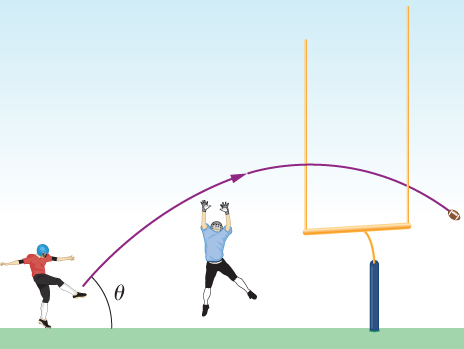A truck is traveling east at 80 km/h. At an intersection 32 km ahead, a car is traveling north at 50 km/h. (a) How long after this moment will the vehicles be closest to each other? (b) How far apart will they be at that point?

### Glossary

reference frame
coordinate system in which the position, velocity, and acceleration of an object at rest or moving is measured
relative velocity
velocity of an object as observed from a particular reference frame, or the velocity of one reference frame with respect to another reference frame